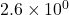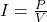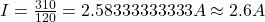## How much current does a 310 W device draw when given a voltage of 120 V? – 3.6•10^4 A – 4.0•10^-2 A –

Question

How much current does a 310 W device draw when given a voltage of 120 V?

– 3.6•10^4 A

– 4.0•10^-2 A

– 4.2•10^2 A

– 2.6•10^0 A

in progress 0
2 months 2021-08-03T20:21:31+00:00 1 Answers 12 views 0Explanation:

Power is expressed as rhe product of voltage and current hence P=IV where V is voltage across the circuit, I is current and P is power. Making current the subject of rhe formula thenSubstituting 310 W for power and 120 V for voltage thenGiving the answer in scientific notation, then current is